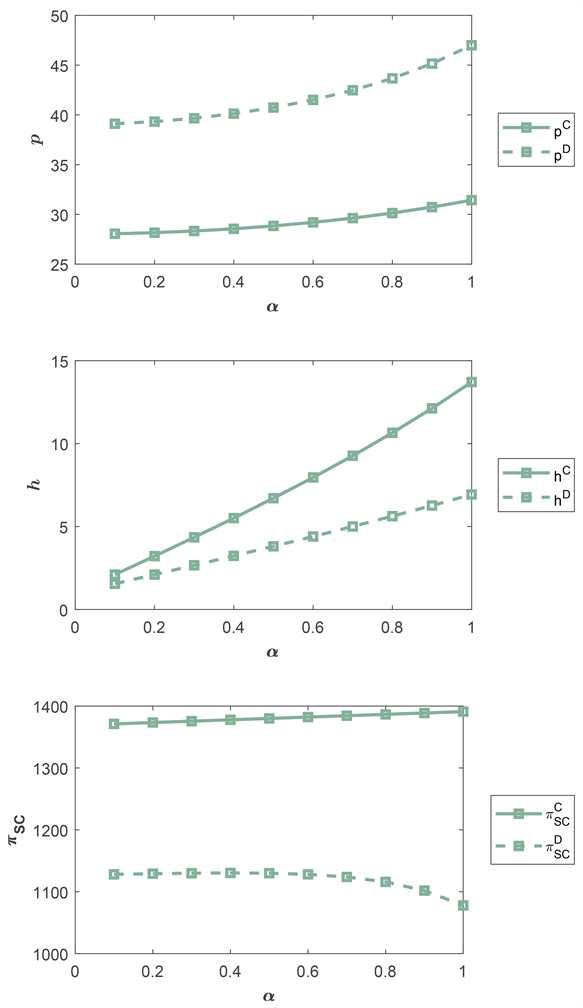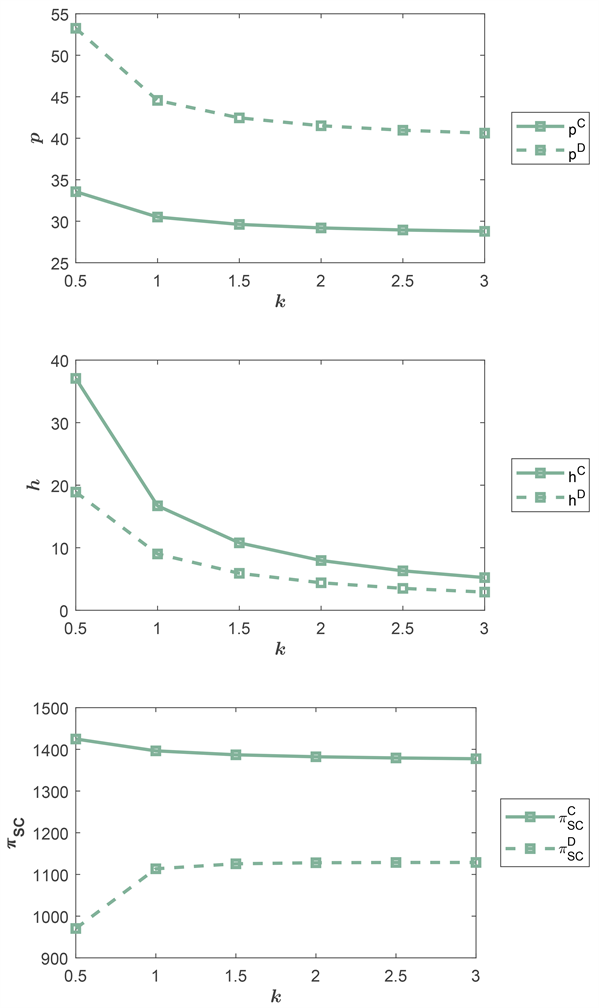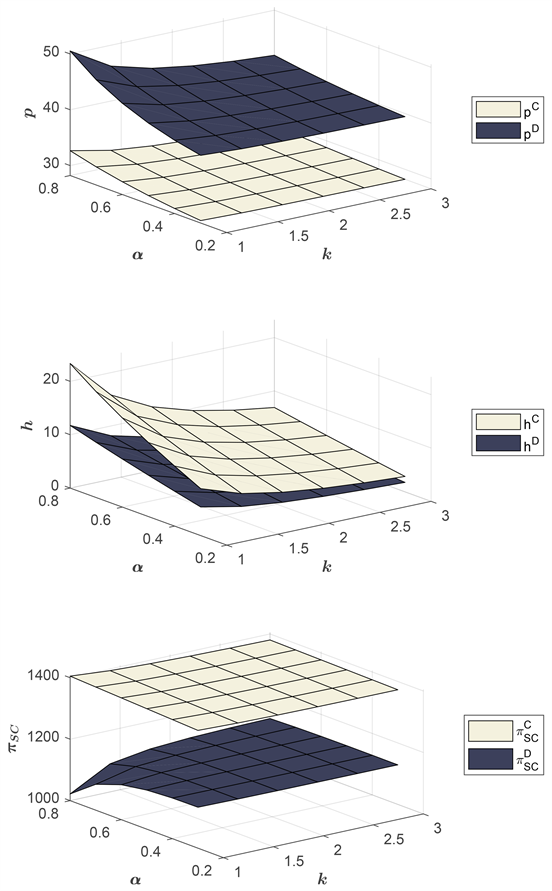#### 期刊菜单

Research on Innovative Decision-Making Game of Supply Chain Emission Reduction Based on Cap-and-Trade Policy

Abstract: In the face of the increasingly severe global climate situation, supply chain decarbonization has gradually become a topic of concern and future development trend. In this context, based on the supply chain theory and game theory, this paper constructs a game model of supply chain innovation decision based on the background of carbon cap and carbon trading policy, and analyzes the low-carbon performance of both suppliers under the centralized and decentralized decision situations. It is found that the centralized decision is better than the decentralized decision in all dimensions, and the magnitude of low-carbon innovation cost and the degree of consumers’ low-carbon preference for products play different directions in the two decision models.

1. 引言

2. 问题描述与参数设定3. 供应链集中决策模型(C)

$\mathrm{max}{\pi }_{SC}^{C}\left(h,p\right)=\left(p-c\right)Q-\frac{1}{2}k{h}^{2}-{p}_{e}\left({e}_{0}Q-G-h\right)$

${h}^{C}=\frac{2b{p}_{e}-\alpha \left(b\left({e}_{0}{p}_{e}+c\right)-a\right)}{2bk-{\alpha }^{2}}$

${p}^{C}=\frac{\alpha {p}_{e}+k\left(b{e}_{0}{p}_{e}+bc+a\right)-{\alpha }^{2}\left({e}_{0}{p}_{e}+c\right)}{2bk-{\alpha }^{2}}$

${Q}^{C}=\frac{2\alpha {p}_{e}-k\left(b\left({e}_{0}{p}_{e}+c\right)-a\right)}{2k}$

4. 供应链分散决策模型(D)

$\mathrm{max}{\pi }_{R}^{D}\left(p\right)=\left(p-w\right)\left(a-bp+\alpha h\right)$

$p=\frac{\alpha h+wb+a}{2b}$

$\mathrm{max}{\pi }_{M}^{D}\left(h,w\right)=\left(w-c\right)Q-\frac{1}{2}k{h}^{2}-{p}_{e}\left({e}_{0}Q-G-h\right)$

$Q=a-bp+\alpha h$

${h}^{D}=\frac{2b{p}_{e}-\alpha \left(b\left({e}_{0}{p}_{e}+c\right)-a\right)}{2bk-2{\alpha }^{2}}$

${w}^{D}=\frac{2\alpha {p}_{e}+k\left(b{e}_{0}{p}_{e}+bc+a\right)-2{\alpha }^{2}\left({e}_{0}{p}_{e}+c\right)}{2bk-2{\alpha }^{2}}$

${p}^{D}=\frac{b\left({\alpha }^{2}\left(-3{e}_{0}{p}_{e}-3c\right)+4\alpha {p}_{e}+3ak\right)+{b}^{2}k\left({e}_{0}{p}_{e}+c\right)-{\alpha }^{2}a}{4b\left(bk-{\alpha }^{2}\right)}$

${Q}^{D}=\frac{a-b\left({e}_{0}{p}_{e}+c\right)}{4}$

5. 算例仿真分析

5.1. 低碳敏感系数变化的影响分析( $k=2$ )

5.2. 低碳创新成本系数变化的影响分析( $\alpha =0.6$ )Figure 1. Influence of low carbon sensitivity coefficient changes on supply chainFigure 2. Influence of change of low-carbon innovation cost coefficient on supply chainTable 2. α changes in retail prices, low-carbon levels of products and supplyTable 3. Influence of k changes on retail price, low carbon level of products and profit of supply chain

6. 结果讨论Figure 3. Influence of common changes of low-carbon sensitivity coefficient and low-carbon innovation cost coefficient

  胡鞍钢, 鄢一龙, 张君忆, 高宇宁, 刘生龙. 中国碳排放趋势: 从相对减排到绝对减排(1990-2050年) [C]//清华大学国情研究中心. 国情报告第十八卷2015年. 北京: 清华大学国情研究中心, 2017: 550-567.  周泽辉, 张桂涛, 尹晓娜. 低碳背景下政府、企业与低碳服务提供商的演化博弈[J/OL]. 运筹与管理. https://kns.cnki.net/kcms/detail/34.1133.G3.20220321.2019.004.html  Bai, Q., Gong, Y., Jin, M., et al. (2019) Effects of Carbon Emission Reduction on Supply Chain Coordination with Vendor-Managed Deteriorating Product Inventory. International Journal of Production Economics, 208, 83-99. https://doi.org/10.1016/j.ijpe.2018.11.008  邬彩霞. 中国低碳经济发展的协同效应研究[J]. 管理世界, 2021, 37(8): 105-117.  徐建中, 贾大风, 李奉书, 王玥. 装备制造企业低碳技术创新对企业绩效的影响研究[J]. , 2018, 30(3): 82-94.  Li, F., Xu, X., Li, Z., et al. (2021) Can Low-Carbon Technological Innovation Truly Im-prove Enterprise Performance? The Case of Chinese Manufacturing Companies. Journal of Cleaner Production, 293, Article ID: 125949. https://doi.org/10.1016/j.jclepro.2021.125949  Zakeri, I.A., Dehghanian, F., Fahimnia, B., et al. (2015) Carbon Pricing versus Emissions Trading: A Supply Chain Planning Perspective. International Journal of Production Economics, 164, 197-205. https://doi.org/10.1016/j.ijpe.2014.11.012  Chai, Q.F., Xiao, Z.D., Lai, K.H., et al. (2018) Can Carbon Cap and Trade Mechanism Be Beneficial for Remanufacturing? International Journal of Production Economics, 203, 311-321. https://doi.org/10.1016/j.ijpe.2018.07.004  Zhao, R., Zhou, X., Han, J.J. and Liu, C.L. (2016) For the Sustainable Performance of the Carbon Reduction Labeling Policies under an Evolutionary Game Simulation. Technological Forecasting and Social Change, 112, 262-274. https://doi.org/10.1016/j.techfore.2016.03.008  李友东, 赵道致, 夏良杰. 低碳供应链纵向减排合作下的政府补贴策略[J]. 运筹与管理, 2014, 23(4): 1-11.  Li, H., Wang, C.X., Shang, M., et al. (2019) Cooperative Decision in a Closed-Loop Supply Chain Considering Carbon Emission Reduction and Low-Carbon Promotion. Environmental Progress & Sustainable Energy, 38, 143-153. https://doi.org/10.1002/ep.13092  王一雷, 朱庆华, 夏西强. 基于消费偏好的供应链上下游联合减排协调契约博弈模型[J]. 系统工程学报, 2017, 32(2): 188-198.  王冬冬, 刘勇. 考虑利他偏好和碳减排努力绩效的供应链决策[J]. 工业工程与管理, 2020, 25(6): 116-125.  赵丹, 戢守峰. 公平关切和低碳偏好下供应链减排投资策略研究[J]. 工业技术经济, 2020, 39(1): 94-104.  Benjaafar, S., Li, Y.Z. and Daskin, M. (2013) Carbon Footprint and the Management of Supply Chains: Insights from Simple Models. IEEE Transactions on Automation Science and Engineering, 10, 99-116. https://doi.org/10.1109/TASE.2012.2203304  叶同, 关志民, 张大儒, 曲优. Nash讨价还价公平关切下基于低碳商誉的供应链联合减排与广告的动态优化与协调[J]. 中国管理科学, 2021, 29(3): 119-132.  张令荣, 王健, 彭博. 内外部碳配额交易路径下供应链减排决策研究[J]. 中国管理科学, 2020, 28(11): 145-154.  刘枚莲, 李宗活, 张婕. 基于前景理论的政企低碳策略演化博弈分析[J]. 科技管理研究, 2017, 37(20): 245-253.  D’Aspremont, C. and Jacquemin, A. (1998) Cooperative and Non-Cooperative R&D in Duopoly with Spillovers. America Economic Review, 78, 1133-1137.  王芹鹏, 赵道致, 何龙飞. 供应链企业碳减排投资策略选择与行为演化研究[J]. 管理工程学报, 2014, 28(3): 181- 189+180.  Zhu, W. and He, Y. (2017) Green Product Design in Supply Chains under Competition. European Journal of Operational Research, 258, 165-180. https://doi.org/10.1016/j.ejor.2016.08.053  Ghosh, D. and Shah, J. (2015) Supply Chain Analysis under Green Sensitive Consumer Demand and Cost Sharing Contract. International Journal of Production Economics, 164, 319-329. https://doi.org/10.1016/j.ijpe.2014.11.005  刘丛, 黄卫来, 杨超, 等. 竞争环境下制造商激励共享供应商创新的决策研究[J]. 系统工程学报, 2020, 35(1): 105- 119.  杨光勇, 计国君. 构建基于产品生命周期的低碳足迹供应链[J]. 厦门大学学报(哲学社会科学版), 2013(2): 65-74.  骆瑞玲, 范体军, 夏海洋. 碳排放交易政策下供应链碳减排技术投资的博弈分析[J]. 中国管理科学, 2014, 22(11): 44-53.